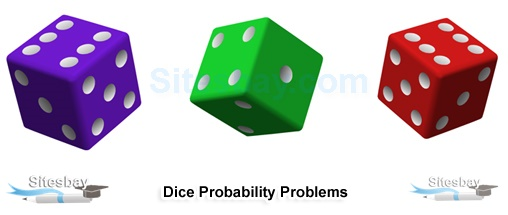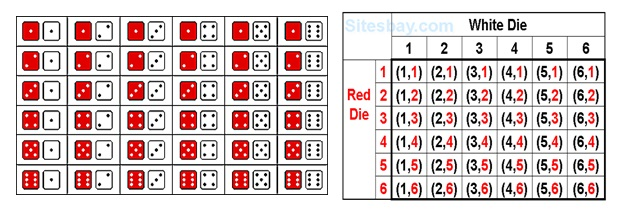# Dice Probability Problems

## Dice Probability Problems

The dice used here is the one used to play Ludo. A typical dice has numbers 1, 2, 3, 4, 5 and 6 are written over its six faces.One popular way to study probability is to roll dice. A standard die has six sides printed with little dots numbering 1, 2, 3, 4, 5, and 6. If the die is fair (and we will assume that all of them are), then each of these outcomes is equally likely. Since there are six possible outcomes, the probability of obtaining any side of the die is 1/6. The probability of rolling a 1 is 1/6, the probability of rolling a 2 is 1/6, and so on.

When a single die is thrown, there are six possible outcomes: 1, 2, 3, 4, 5, 6. The probability of any one of them is 1/6 of rolling a 1 is 1/6, the probability of rolling a 2 is 1/6, and so on. But what happens if we add another die? What are the probabilities for rolling two dice?

### Dice Roll Probability

To correctly determine the probability of a dice roll, we need to know two things:

• The size of the sample space or the set of total possible outcomes
• How often an event occursIn probability, an event is a certain subset of the sample space. For example, when only one die is rolled, as in the example above, the sample space is equal to all of the values on the die, or the set (1, 2, 3, 4, 5, 6). Since the die is fair, each number in the set occurs only once. In other words, the frequency of each number is 1. To determine the probability of rolling any one of the numbers on the die, we divide the event frequency (1) by the size of the sample space (6), resulting in a probability of 1/6.

Rolling two fair dice more than doubles the difficulty of calculating probabilities. This is because rolling one die is independent of rolling a second one. One roll has no effect on the other. When dealing with independent events we use the multiplication rule. The use of a tree diagram demonstrates that there are 6 x 6 = 36 possible outcomes from rolling two dice.

Suppose that the first die we roll comes up as a 1. The other die roll could be a 1, 2, 3, 4, 5, or 6. Now suppose that the first die is a 2. The other die roll again could be a 1, 2, 3, 4, 5, or 6. We have already found 12 potential outcomes, and have yet to exhaust all of the possibilities of the first die.

### Probability Table of Rolling Two Dice

The possible outcomes of rolling two dice are represented in the table below. Note that the number of total possible outcomes is equal to the sample space of the first die (6) multiplied by the sample space of the second die (6), which is 36.

123456
1(1, 1)(1, 2)(1, 3)(1, 4)(1, 5)(1, 6)
2(2, 1)(2, 2)(2, 3)(2, 4)(2, 5)(2, 6)
3(3, 1)(3, 2)(3, 3)(3, 4(3, 5)(3, 6)
4(4, 1)(4, 2)(4, 3)(4, 4)(4, 5)(4, 6)
5(5, 1)(5, 2)(5, 3)(5, 4)(5, 5)(5, 6)
6(6, 1)(6, 2)(6, 3)(6, 4)(6, 5)(6, 6)

### Three or More Dice

The same principle applies if we are working on problems involving three dice. We multiply and see that there are 6 x 6 x 6 = 216 possible outcomes. As it gets cumbersome to write the repeated multiplication, we can use exponents to simplify work. For two dice, there are 6^2 possible outcomes. For three dice, there are 6^3 possible outcomes. In general, if we roll n dice, then there are a total of 6^n possible outcomes.

When a single die is thrown, there are six possible outcomes: 1, 2, 3, 4, 5, 6. The probability of any one of them is 1/6.

## Formula

```Probability of an event happening =  Number of ways it can happen / Total number of outcomes
```

Example question: What is the probability of rolling a 4 or 7 for two 6 sided dice?

In order to know what the odds are of rolling a 4 or a 7 from a set of two dice, you first need to find out all the possible combinations. You could roll a double one , or a one and a two . In fact, there are 36 possible combinations.

### Dice Rolling Probability: Steps

Step 1: Write out your sample space (i.e. all of the possible results). For two dice, the 36 different possibilities are;

## Formula

```, , , , , ,
, , , , , ,
, , , , , ,
, , , , , ,
, , , , , ,
, , , , , .
```

Step 2: Look at your sample space and find how many add up to 4 or 7 (because we're looking for the probability of rolling one of those numbers). The rolls that add up to 4 or 7 are in bold:

## Formula

```, , , , , ,
, , , ,, ,
, , , , , ,
, , , , , ,
, , , , , ,
, , , , , .
```

There are 9 possible combinations.

Step 3: Take the answer from step 2, and divide it by the size of your total sample space from step 1. What I mean by the "size of your sample space" is just all of the possible combinations you listed. In this case, Step 1 had 36 possibilities, so:

## Formula

```9 / 36 = .25
```